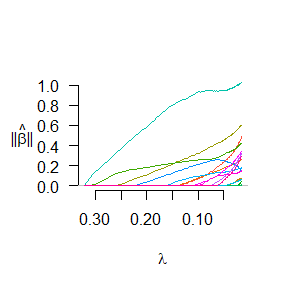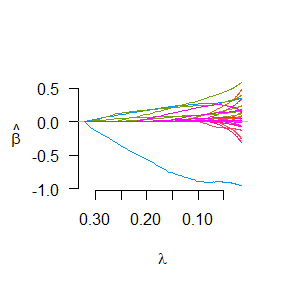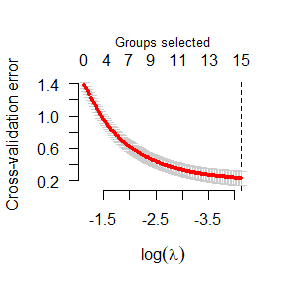# 1 Introduction

PCLassoReg is a package that implements protein complex-based group regression models (PCLasso and PCLasso2) for risk protein complex identification.

## 1.1 PCLasso

PCLasso is a prognostic model that identifies risk protein complexes associated with survival. It has three inputs: a gene/protein expression matrix, survival data, and protein complexes. PCLasso is based on the Cox PH model and estimates the Cox regression coefficients by maximizing partial likelihood with regularization penalty. Considering that genes usually function by forming protein complexes, PCLasso regards genes belonging to the same protein complex as a group, and constructs a l1/l2 penalty based on the sum (i.e., l1 norm) of the l2 norms of the regression coefficients of the group members to perform the selection of features at the group level. It deals with the overlapping problem of protein complexes by constructing a latent group Lasso-Cox model. Through the final sparse solution, we can predict the patient’s risk score based on a small set of protein complexes and identify risk protein complexes that are frequently selected to construct prognostic models. The penalty parameters “grSCAD” and “grMCP” can also be used to identify survival-associated risk protein complexes. Their penalty for large coefficients is smaller than “grLasso”, so they tend to choose less risk protein complexes.

PCLasso solves the following problem: \begin{equation} \begin{aligned} & \hat\beta = \mathop{\arg\min}_{\beta\in{R}^p}\left[-\frac{2}{n}\sum_{r=1}^{m}\left(x_{j(r)}^ {T}\mathbf{\beta}-\log\bigg(\sum_{j\in{R}_r}e^{x_{i}^{T}\beta}\bigg)\right)+ \lambda\sum_{k=1}^{K}\sqrt{|G_k|}\left\|\gamma_k\right\|\right]\\ & \mathrm{s.t.}\ \ \beta=\sum_{k=1}^{K}\gamma_k \end{aligned} \end{equation} where the first term represents the log partial likelihood function, and the second term is a group Lasso (“grLasso”) penalty.

## 1.2 PCLasso2

PCLasso2 is a classification model that identifies risk protein complexes associated with classes. It has three inputs: a gene/protein expression matrix, a vector of binary response variables, and a number of known protein complexes. PCLasso2 is based on the logistic regression model and estimates the logistic regression coefficients by maximizing likelihood function with regularization penalty. PCLasso2 regards proteins belonging to the same protein complex as a group and constructs a group Lasso penalty (l1/l2 penalty) based on the sum (i.e. l1 norm) of the l2 norms of the regression coefficients of the group members to perform the selection of features at the group level. With the group Lasso penalty, PCLasso2 trains the logistic regression model and obtains a sparse solution at the protein complex level, that is, the proteins belonging to a protein complex are either wholly included or wholly excluded from the model. PCLasso2 outputs a prediction model and a small set of protein complexes included in the model, which are referred to as risk protein complexes. The PCSCAD and PCMCP are performed by setting the penalty parameter as “grSCAD” and “grMCP”, respectively.

PCLasso2 solves the following problem: \begin{equation} \begin{aligned} & \mathop{\arg\min}_{\beta_0,\beta}\left\{-\frac{1}{n}\sum_{i=1}^{n} \left[y_i\left(\beta_0+x_i^{T}\beta\right)-\log\bigg(1+e^{\beta_0+ x_{i}^{T}\beta}\bigg)\right]+ \lambda\sum_{k=1}^{K}\sqrt{|G_k|}\left\|\gamma_k\right\|\right\}\\ & \mathrm{s.t.}\ \ \beta=\sum_{k=1}^{K}\gamma_k \end{aligned} \end{equation} where the first term represents the log-likelihood function, and the second term is a group Lasso (“grLasso”) penalty.

# 2 Installation

Like many other R packages, the simplest way to obtain RLassoCox is to install it directly from CRAN. Type the following command in R console:

install.packages("PCLassoReg")

To install the latest development version from GitHub:

devtools::install_github("weiliu123/PCLassoReg")

# 3 PCLassoReg

In this section, we will go over the main functions, see the basic operations and have a look at the outputs. Users may have a better idea after this section what functions are available, which one to choose, or at least where to seek help.

First, we load the PCLassoReg package:

library("PCLassoReg")

## 3.1 PCLasso

The PCLasso model accepts a gene/protein expression matrix, survival data, and protein complexes for training the prognostic model. We load a set of data created beforehand for illustration. Users can either load their own data or use those saved in the workspace.

# load data
data(survivalData)
data(PCGroups)

x <- survivalData$Exp y <- survivalData$survData

The commands load a list survivalData that contains a gene expression matrix Exp and survival information survData of patients in Exp, and a data frame PCGroups containing the protein complexes downloaded from [CORUM] (https://mips.helmholtz-muenchen.de/corum/).

survData is an n x 2 matrix, with a column “time” of failure/censoring times, and “status” a 0/1 indicator, with 1 meaning the time is a failure time, and zero a censoring time.

y <- classData$Label The commands load a list classData that contains a protein expression matrix Exp and class labels Label of patients in Exp, and a data frame PCGroups containing the protein complexes downloaded from [CORUM] (https://mips.helmholtz-muenchen.de/corum/). Use getPCGroups function to get human protein complexes from PCGroups. Note that the parameter Type should be consistent the gene names in Exp. # get human protein complexes PC.Human <- getPCGroups(Groups = PCGroups, Organism = "Human", Type = "GeneSymbol") In order to train and test the predictive performance of the PCLasso2 model, we divide the data set into a training set and a test set. set.seed(20150122) idx.train <- sample(nrow(x), round(nrow(x)*2/3)) x.train <- x[idx.train,] y.train <- y[idx.train] x.test <- x[-idx.train,] y.test <- y[-idx.train] We usually use cv.PCLasso2 instead of PCLasso2 to train the model, because cv.PCLasso2 helps us choose the best $$\lambda$$ through k-fold cross validation. Train the PCLasso2 model based on the training set data: cv.fit2 <- cv.PCLasso2(x = x.train, y = y.train, group = PC.Human, penalty = "grLasso", family = "binomial", nfolds = 10) cv.fit2 contains a list object that includes a cv.grpreg object cv.fit and a list of detected protein complexes complexes.dt. complexes.dt contains the proteins that exist in the expression matrix x.train and are used for model training. We can visualize the norm of the protein complexes by executing the plot function: # plot the norm of each group plot(cv.fit2, norm = TRUE)Each curve in the figure corresponds to a group (protein complex). It shows the path of the norm of each protein complex and $$L_1$$-norm when $$\lambda$$ varies. Visualize the coefficients: # plot the individual coefficients plot(cv.fit2, norm = FALSE)Each curve in the figure corresponds to a variable (gene/protein). It shows the path of the coefficient of each gene/protein and $$L_1$$-norm when $$\lambda$$ varies. The optimal $$\lambda$$ value and a cross validated error plot can be obtained to help evaluate our model. # plot the cross-validation error (deviance) plot(cv.fit2, type = "cve")In this plot, the vertical line shows where the cross-validation error curve hits its minimum. The optimal $$\lambda$$ can be obtained: cv.fit2$cv.fit$lambda.min #>  0.01601148 We can check the selected protein complexes (risk protein complexes) in our model. # Selected protein complexes at lambda.min sel.groups <- predict(object = cv.fit2, type="groups", lambda = cv.fit2$cv.fit$lambda.min) # For values of lambda not in the sequence of fitted models, linear # interpolation is used. sel.groups <- predict(object = cv.fit2, type="groups", lambda = c(0.1, 0.05)) Check the number of risk protein complexes: # The number of risk protein complexes at lambda.min sel.ngroups <- predict(object = cv.fit2, type="ngroups", lambda = cv.fit2$cv.fit$lambda.min) # For values of lambda not in the sequence of fitted models, linear # interpolation is used. sel.ngroups <- predict(object = cv.fit2, type="ngroups", lambda = c(0.1, 0.05)) Check the norms of the protein complexes: # The coefficients of protein complexes at lambda.min groups.norm <- predict(object = cv.fit2, type="coefficients", lambda = cv.fit2$cv.fit$lambda.min) # For values of lambda not in the sequence of fitted models, linear # interpolation is used. groups.norm <- predict(object = cv.fit2, type="coefficients", lambda = c(0.1, 0.05)) Check the selected covariates (risk individual genes/proteins) in our model: # Selected genes/proteins at lambda.min sel.vars <- predict(object = cv.fit2, type="vars", lambda=cv.fit2$cv.fit$lambda.min) # For values of lambda not in the sequence of fitted models, linear # interpolation is used. sel.vars <- predict(object = cv.fit2, type="vars", lambda=c(0.1, 0.05)) Check the number of risk individual genes/proteins: # The number of risk genes/proteins at lambda.min sel.nvars <- predict(object = cv.fit2, type="nvars", lambda=cv.fit2$cv.fit$lambda.min) # For values of lambda not in the sequence of fitted models, linear # interpolation is used. sel.vars <- predict(object = cv.fit2, type="nvars", lambda=c(0.1, 0.05)) Due to the overlap of protein complexes, there may be duplicates in the above risk genes/proteins. Use the following command to remove duplication: # Selected genes/proteins at lambda.min sel.vars <- predict(object = cv.fit2, type="vars.unique", lambda=cv.fit2$cv.fit$lambda.min) # For values of lambda not in the sequence of fitted models, linear # interpolation is used. sel.vars <- predict(object = cv.fit2, type="vars.unique", lambda=c(0.1, 0.05)) # The number of risk genes/proteins at lambda.min sel.nvars <- predict(object = cv.fit2, type="nvars.unique", lambda=cv.fit2$cv.fit$lambda.min) # For values of lambda not in the sequence of fitted models, linear # interpolation is used. sel.vars <- predict(object = cv.fit2, type="nvars.unique", lambda=c(0.1, 0.05)) The fitted PCLasso2 model can by used to predict the probability that the sample is a tumor sample: # predict probabilities of samples in x.test s <- predict(object = cv.fit2, x = x.test, type="response", lambda=cv.fit2$cv.fit$lambda.min) # For values of lambda not in the sequence of fitted models, linear # interpolation is used. s <- predict(object = cv.fit2, x = x.test, type="response", lambda=c(0.1, 0.05)) Predict the class labels of new samples: # predict class labels of samples in x.test s <- predict(object = cv.fit2, x = x.test, type="class", lambda=cv.fit2$cv.fit$lambda.min) # For values of lambda not in the sequence of fitted models, linear # interpolation is used. s <- predict(object = cv.fit2, x = x.test, type="class", lambda=c(0.1, 0.05)) ## 3.3 Other penalties In addition to “grLasso”, two other penalty functions “grSCAD” and “grMCP” can be used to train PCLasso and PCLasso2 models. Their penalty for large coefficients is smaller than “grLasso”, so they tend to choose less risk protein complexes. Note that the two penalty functions have a new parameter gamma. Train the PCLasso model: # load data data(survivalData) data(PCGroups) x = survivalData$Exp
y = survivalData$survData PC.Human <- getPCGroups(Groups = PCGroups, Organism = "Human", Type = "EntrezID") # fit PCSCAD model fit.PCSCAD <- PCLasso(x, y, group = PC.Human, penalty = "grSCAD", gamma = 6) # fit PCMCP model fit.PCMCP <- PCLasso(x, y, group = PC.Human, penalty = "grMCP", gamma = 5) Train the PCLasso2 model: # load data data(classData) data(PCGroups) x = classData$Exp
y = classData\$Label

PC.Human <- getPCGroups(Groups = PCGroups, Organism = "Human",
Type = "GeneSymbol")

family = "binomial", gamma = 10)

# fit PCMCP model
fit.PCMCP2 <- PCLasso2(x, y, group = PC.Human, penalty = "grMCP",
family = "binomial", gamma = 9)

Other functions are similar to PCLasso and PCLasso2 models.

# 4 Reference

• PCLasso2: a protein complex-based, group Lasso-logistic model for risk protein complex discovery. To be published.

• PCLasso: a protein complex-based, group lasso-Cox model for accurate prognosis and risk protein complex discovery. Brief Bioinform, 2021.

• Park, H., Niida, A., Miyano, S. and Imoto, S. (2015) Sparse overlapping group lasso for integrative multi-omics analysis. Journal of computational biology: a journal of computational molecular cell biology, 22, 73-84.